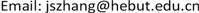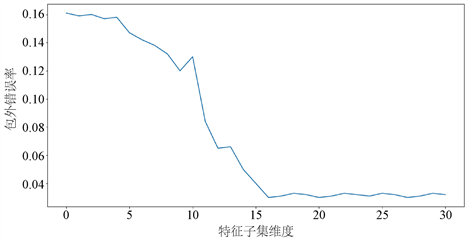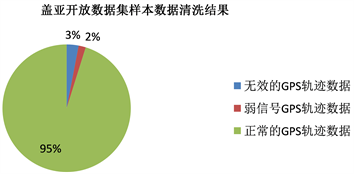﻿ 基于随机森林算法的盖亚大数据清洗的研究 An Automatic Data Cleaning Method for GPS Trajectory Data on Didi Chuxing GAIA Open Dataset Using Random Forest Algorithm

Computer Science and Application
Vol. 09  No. 09 ( 2019 ), Article ID: 32255 , 6 pages
10.12677/CSA.2019.99196

An Automatic Data Cleaning Method for GPS Trajectory Data on Didi Chuxing GAIA Open Dataset Using Random Forest Algorithm

Jiashun Zhang

Department of Transportation, Hebei University of Technology, TianjinReceived: Aug. 29th, 2019; accepted: Sep. 13th, 2019; published: Sep. 20th, 2019ABSTRACT

A new data cleaning method for the GPS trajectory data on Didi Chuxing GAIA Open Dataset is developed. Random forests algorithm is employed to the identification of invalid, weak, and normal data of the Didi Chuxing GAIA Open Dataset raw data. Firstly, the feature set is selected according to the mathematical characteristics of three types of data, and then the optimal feature subset dimension is determined. Finally, to implement the proposed method, the Pandas and scikit-learn Python library are used to read and process the data and the result illustrates the effectiveness of this method.

Keywords:Data Cleaning, Machine Learning, Random ForestCopyright © 2019 by author(s) and Hans Publishers Inc.1. 引言

Jennifer Baur  提出了一个有效的检测缺失数据并改良数据的方法。Patrick Röhm  针对投资数据设计了一种识别企业风险投资者的数据清理程序。Tomer Gueta  提出了用于量化用户级大数据清理价值的分布式模型。Ridha Khedri  则设计了一种基于代数的数据清洗方法。Salem  提出了基于条件函数依赖的数据清理规则。Saul Gilla  针对数据流的清洗设计了一个分布式的计算框架。

2. 滴滴出行盖亚开放数据集Table 1. The fields of GPS trajectory data of Didi Chuxing GAIA Open DatasetTable 2. The fields of order data of Didi Chuxing GAIA Open DatasetFigure 1. Typical patterns of vehicle speed from raw Didi Chuxing GAIA Open Dataset

3. 盖亚轨迹数据集的自动清洗方法

$\begin{array}{c}{l}_{i}=12756274\\ ×\mathrm{arcsin}\left(\sqrt{{\mathrm{sin}}^{2}\left(\frac{\text{π}×\left(lat{i}_{i}-lat{i}_{i-1}\right)}{360}\right)+\mathrm{cos}\left(\frac{\text{π}×lat{i}_{i}}{180}\right)×\mathrm{cos}\left(\frac{\text{π}×lat{i}_{i-1}}{180}\right)×{\mathrm{sin}}^{2}\left(\frac{\text{π}×\left(lon{g}_{i}-lon{g}_{i-1}\right)}{360}\right)}\right)\end{array}$ (1)

$i=1,2,\cdots ,n$ ，其中 $lat{i}_{i}$$lon{g}_{i}$ 分别是点i在GCJ-02坐标系下的经度和纬度坐标， ${t}_{i}$ 车辆在i时的时间，其数据为unix时间戳格式。

${v}_{i}=\frac{{l}_{i}-{l}_{i-1}}{{t}_{i}-{t}_{i-1}}$ (2)

${a}_{i}=\frac{{v}_{i}-{v}_{i-1}}{{t}_{i}-{t}_{i-1}}$ (3)

$i=1,2,\cdots ,n$

${v}_{ij}=\frac{{l}_{i}-{l}_{i-j}}{{t}_{i}-{t}_{i-j}}$(4)

$j=1,2,\cdots ,M-1$Figure 2. The influences of the number of feature variables on the out-of-bag classification errorFigure 3. The results of the automatic cleaning of Sample Data Set

4. 结论

An Automatic Data Cleaning Method for GPS Trajectory Data on Didi Chuxing GAIA Open Dataset Using Random Forest Algorithm[J]. 计算机科学与应用, 2019, 09(09): 1747-1752. https://doi.org/10.12677/CSA.2019.99196

1. 1. Baur, J., Moreno-Villanueva, M., Kötter, T., Sindlinger, T., Bürkle, A., Berthold, M.R. and Junk, M. (2015) MARK-AGE Data Management: Cleaning, Exploration and Visualization of Data. Mechanisms of Ageing and Develop-ment, 151, 38-44. https://doi.org/10.1016/j.mad.2015.05.007

2. 2. Röhm, P., Merz, M. and Kuckertz, A. (2019) Identifying Corporate Venture Capital Investors—A Data-Cleaning Procedure. Finance Research Letters.

3. 3. Gueta, T. and Carmel, Y. (2016) Quantifying the Value of User-Level Data Cleaning for Big Data: A Case Study Using Mammal Distribution Models. Ecological Informatics, 34, 139-145. https://doi.org/10.1016/j.ecoinf.2016.06.001

4. 4. Khedri, R., Chiang, F. and Sabri, K.E. (2013) An Algebraic Approach towards Data Cleaning. Procedia Computer Science, 21, 50-59. https://doi.org/10.1016/j.procs.2013.09.009

5. 5. Salem, R. and Abdo, A. (2016) Fixing Rules for Data Cleaning Based on Conditional Functional Dependency. Future Computing and Informatics Journal, 1, 10-26. https://doi.org/10.1016/j.fcij.2017.03.002

6. 6. Gilla, S. and Lee, B. (2015) A Framework for Distributed Cleaning of Data Streams. Procedia Computer Science, 52, 1186-1191. https://doi.org/10.1016/j.procs.2015.05.156

7. 7. Li, C., Lan, T., Wang, Y., Liu, J., Xie, J., Lan, T., Li, H. and Qin, H. (2018) An Automatic Data Cleaning Procedure for the Electron Cyclotron Emission Imaging on EAST Tokamak Using Machine Learning Algorithm. Journal of Instrumenta-tion, 13, P10029. https://doi.org/10.1088/1748-0221/13/10/P10029

8. 8. 张西宁, 张雯雯, 周融通, 向宙. 基于单类随机森林的异常检测方法及应用[J/OL]. 西安交通大学学报, 2019(12): 1-8.

9. 9. 徐乔, 张霄, 余绍淮, 陈启浩, 刘修国. 综合多特征的极化SAR图像随机森林分类算法[J]. 遥感学报, 2019, 23(4): 685-694.

10. 10. 郑建华, 刘双印, 贺超波, 符志强. 基于混合采样策略的改进随机森林不平衡数据分类算法[J]. 重庆理工大学学报(自然科学), 2019, 33(7): 113-123.

11. 11. 刘云翔, 陈斌, 周子宜. 一种基于随机森林的改进特征筛选算法[J]. 现代电子技术, 2019, 42(12): 117-121.

12. 12. 尹儒, 门昌骞, 王文剑. 一种模型决策森林算法[J/OL]. 计算机科学与探索, 1-11.

13. 13. 林栢全, 肖菁. 基于矩阵分解与随机森林的多准则推荐算法[J]. 华南师范大学学报(自然科学版), 2019, 51(2): 117-122.

14. 14. 张宸宁, 李国成. 基于BL-SMOTE和随机森林的不平衡数据分类[J]. 北京信息科技大学学报(自然科学版), 2019, 34(2): 23-28.

15. 15. 孙悦, 袁健. 基于Spark的改进随机森林算法[J]. 电子科技, 2019, 32(4): 60-63+67.

16. 16. 董娜, 常建芳, 吴爱国. 基于贝叶斯模型组合的随机森林预测方法[J]. 湖南大学学报(自然科学版), 2019, 46(2): 123-130

17. 17. 朱冰, 李伟男, 汪震, 赵健, 何睿, 韩嘉懿. 基于随机森林的驾驶人驾驶习性辨识策略[J]. 汽车工程, 2019, 41(2): 213-218+224.

18. 18. 关晓蔷, 庞继芳, 梁吉业. 基于类别随机化的随机森林算法[J]. 计算机科学, 2019, 46(2): 196-201.